# Almost-period

(diff) ← Older revision | Latest revision (diff) | Newer revision → (diff)

A concept from the theory of almost-periodic functions (cf. Almost-periodic function); a generalization of the notion of a period. For a uniformly almost-periodic function,, a number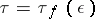is called an-almost-period ofif for all,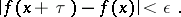For generalized almost-periodic functions the concept of an almost-period is more complicated. For example, in the space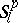an-almost-periodis defined by the inequality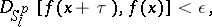whereis the distance between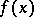andin the metric of.

A set of almost-periods of a functionis said to be relatively dense if there is a number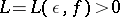such that every interval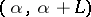of the real line contains at least one number from this set. The concepts of uniformly almost-periodic functions and that of Stepanov almost-periodic functions may be defined by requiring the existence of relatively-dense sets of-almost-periods for these functions.

How to Cite This Entry:
Almost-period. Encyclopedia of Mathematics. URL: http://encyclopediaofmath.org/index.php?title=Almost-period&oldid=32494
This article was adapted from an original article by E.A. Bredikhina (originator), which appeared in Encyclopedia of Mathematics - ISBN 1402006098. See original article